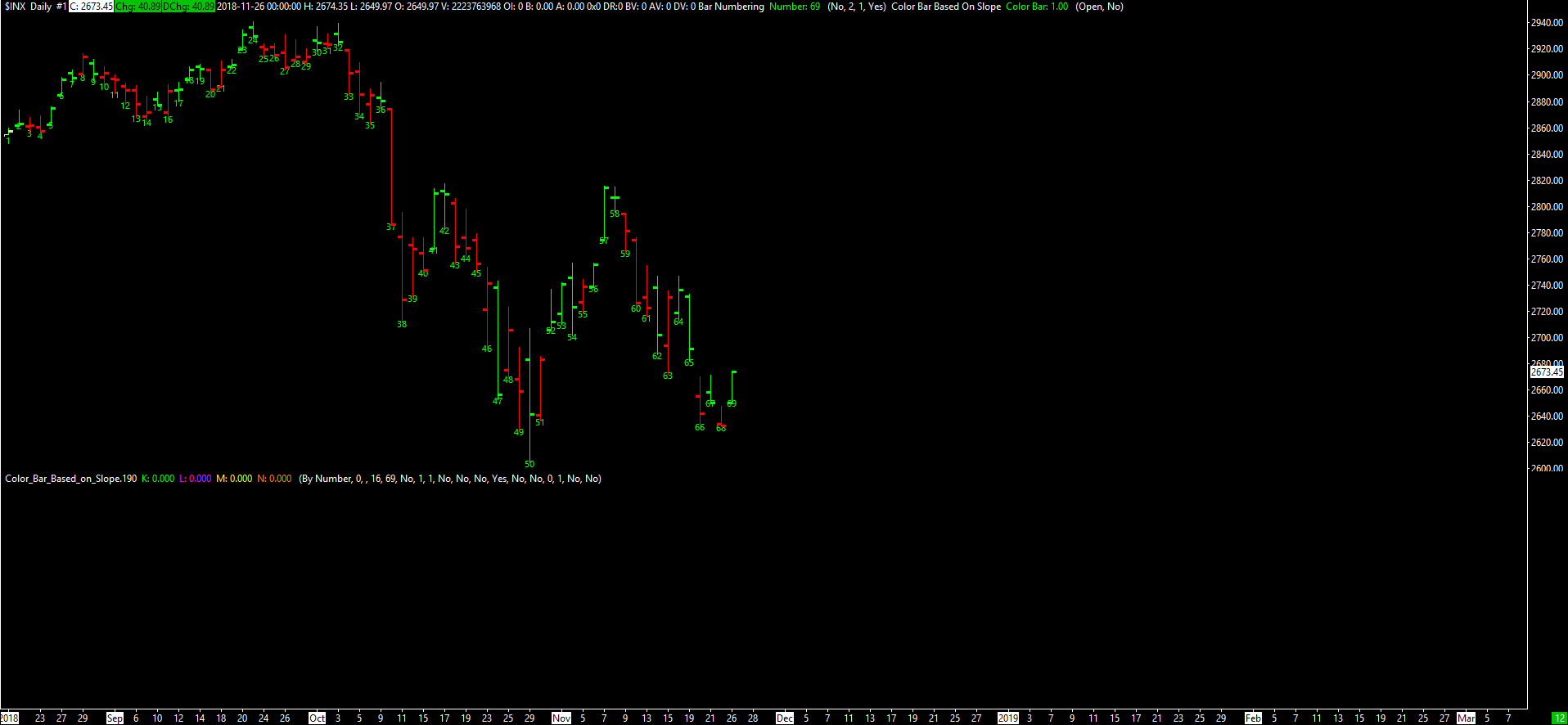# Technical Studies Reference

### Color Bar Based on Slope

This study colors the chart bars according to whether or not the Subgraph of the study that this study is Based On has a positive or negative slope. When Based On is set to <Main Price Graph>, then the main price graph will be colored according to slope. This is useful when using the Line on Close Graph Draw Type.

Let $$X_t$$, $$C_t$$, and $$O_t$$ be the values of the Input Data Input, the Close Price, and the Open Price, respectively, at Index $$t$$. This study colors the chart bar at Index $$t$$ according to the following rules.

• If $$X_t = X_{t - 1}$$, then the study does not color the bar. Instead, the standard coloring is used (that is, green when $$C_t > O_t$$ and red when $$C_t < O_t$$). The standard coloring is automatically used for $$t = 0$$.
• If $$X_t > X_{t - 1}$$, then the entire main price graph bar is colored with the Primary Color of the study (the first color button of the Color Bar Subgraph).
• If $$X_t < X_{t - 1}$$, then the entire main price graph bar is colored with the Secondary Color of the study (the second color button of the Color Bar Subgraph).

For this study to work correctly, the Color Bar Subgraph Draw Style must be set to Color Bar.

If the Use +1 (+slope) and -1 (-slope) for Color Bar Values Input is set to Yes, then the output value is $$1$$ if $$X_t > X_{t - 1}$$, $$0$$ if $$X_t = X_{t - 1}$$, and $$-1$$ if $$X_t < X_{t - 1}$$. If this Input is set to No, then the output value is $$1$$ if $$X_t \neq X_{t - 1}$$ and $$0$$ if $$X_t = X_{t - 1}$$.

#### Inputs

• Input Data: This Input selects the Subgraph from the Based On study that you want to base the coloring on.
• Use +1 (+slope) and -1 (-slope) for Color Bar Values: When the slope is positive, the color bar value will be 1, and when it is negative the color bar value will be -1.